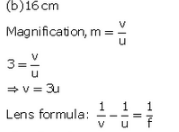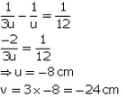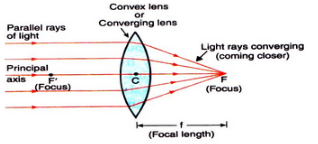Courses

# Solutions of Refraction of Light (Page No- 242) - Physics By Lakhmir Singh, Class 10 Class 10 Notes | EduRev

## Class 10 Physics Solutions By Lakhmir Singh & Manjit Kaur

Created by: Ajay Batra

## Class 10 : Solutions of Refraction of Light (Page No- 242) - Physics By Lakhmir Singh, Class 10 Class 10 Notes | EduRev

The document Solutions of Refraction of Light (Page No- 242) - Physics By Lakhmir Singh, Class 10 Class 10 Notes | EduRev is a part of the Class 10 Course Class 10 Physics Solutions By Lakhmir Singh & Manjit Kaur.
All you need of Class 10 at this link: Class 10

Lakhmir Singh Physics Class 10 Solutions Page No:242

Question 46: A lens of focal length 12 cm forms an erect image three times the size of the object. The distance between the object and image is :
(a) 8 cm
(b) 16 cm
(c) 24 cm
(d) 36 cm

Solution :Distance between object and im age= u-v= -8-(-24) = - 8 + 2 4 = 16 cm

Question 47: If an object is placed 21 cm from a converging lens, the image formed is slightly smaller than the object. If the object is placed 19 cm from the lens, the image formed is slightly larger than the object. The approximate focal length of the lens is :
(a) 5 cm
(b) 10 cm
(c) 18 cm
(d) 20 cm

Solution : (b) 10 cm
The image is slightly smaller than the object when the object lies beyond 2f; and the image is slightly larger than the object when the object between f and 2f. This means that between 21 cm and 19 cm lies 2f. Out of the given options, 20 cm lies between 21 cm and 19 cm.
So, 2f = 20 cm.
f = 10 cm.

Question 48: An object is placed at the following distances from a convex lens of focal length 15 cm :
(a) 35 cm
(b) 30 cm
(c) 20 cm
(d) 10 cm
Which position of the object will produce :
(i) a magnified real image ?
(ii) a magnified virtual image ?
(iii) a diminished real image ?
(iv) an image of same size as the object ?

Solution : Here, f = 15cm and 2f = 30cm
(i) 20 cm (Because a magnified real image is formed when the object is placed between f and 2f).
(ii) 10cm (Because a magnified virtual image is formed when the object is placed between f and the lens).
(iii) 35cm (Because a diminished real image is formed when the object is placed beyonf 2f).
(iv) 30cm (Because an image of same size as the object is formed when the object is placed at 2f).

Question 49: When an object is placed at a distance of 36 cm from a convex lens, an image of the same size as the object is formed. What will be the nature of image formed when the object is placed at a distance of:
(a) 10 cm from the lens ? (b) 20 cm from the lens ?

Solution : Here, 2f = 36 cm, f = 18 cm.
(a) When the object is placed at a distance of 10 cm from the lens, the object lies within the focus. Hence, the image formed is virtual, erect and magnified.
(b) When the object is placed at a distance of 20 cm from the lens, the object lies between f and 2f. Hence, the image formed is real, inverted and magnified.

Question 50: (a) Draw a diagram to show how a converging lens focusses parallel rays of light.
(b) How would you alter the above diagram to show how a converging lens can produce a beam of parallel rays of light.

Solution : (a) A converging lens focusses parallel ray of light as shown below:Figure — A convex lens converges (brings closer) a parallel beam of light rays to a point F on its other side (right side).

(b) Place a source of light at the focus of the converging lens.

Offer running on EduRev: Apply code STAYHOME200 to get INR 200 off on our premium plan EduRev Infinity!

94 docs

,

,

,

,

,

,

,

,

,

,

,

,

,

,

,

,

,

,

,

,

,

,

,

,

;